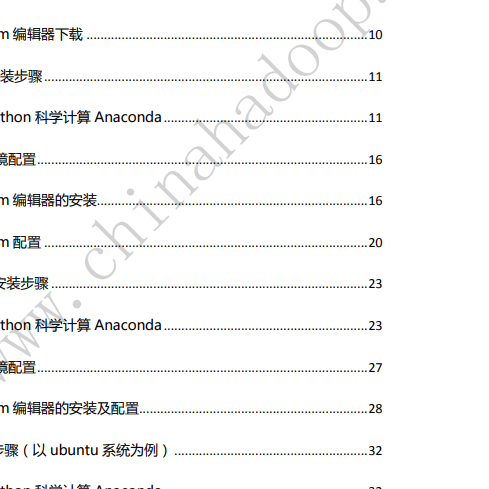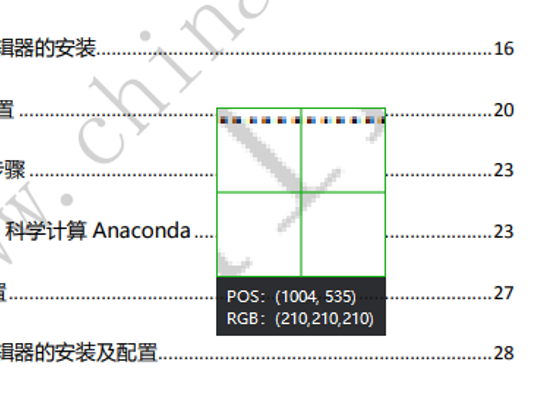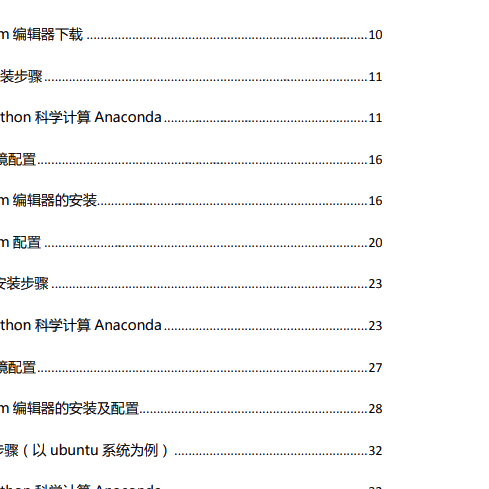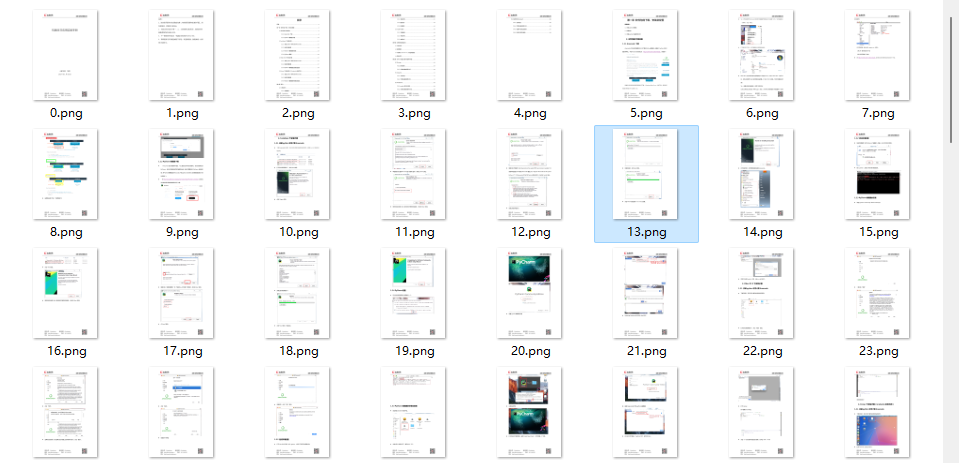网上下载的 pdf 学习资料有一些会带有水印，非常影响阅读。比如下面的图片就是在 pdf 文件上截取出来的。### 安装模块

PIL：Python Imaging Library 是 python 上非常强大的图像处理标准库，但是只能支持 python 2.7，于是就有志愿者在 PIL 的基础上创建了支持 python 3的 pillow，并加入了一些新的特性。

 `1` ``````pip install pillow ``````

pymupdf 可以用 python 访问扩展名为.pdf、.xps、.oxps、.epub、.cbz或.fb2的文件。还支持了许多流行的图像格式，包括多页TIFF图像。

 `1` ``````pip install PyMuPDF ``````

 `1234` ``````from PIL import Image from itertools import product import fitz import os ``````

### 获取图片的 RGB

pdf 去水印的原理和图片去水印的原理差不多，小编先从去除上面那张图片的水印开始。

 `123456789` ``````def remove_img(): image_file = input("请输入图片地址：") img = Image.open(image_file) width, height = img.size for pos in product(range(width), range(height)): rgb = img.getpixel(pos)[:3] print(rgb) ``````

### 图片去水印`12345` ``````rgb = img.getpixel(pos)[:3] if(sum(rgb) >= 620): img.putpixel(pos, (255, 255, 255)) img.save('d:/qsy.png') ``````### PDF 去水印

PDF 去水印的原理和图片去水印的原理大致相同，用 PyMuPDF 打开 pdf 文件后，将 pdf 的每一页都转换为图片 pixmap，pixmap 有它自己的 RGB，只需要将 pdf 水印中的 RGB 改为(255, 255, 255) 最后保存为图片。

 `12345678910111213` ``````def remove_pdf(): page_num = 0 pdf_file = input("请输入 pdf 地址：") pdf = fitz.open(pdf_file); for page in pdf: pixmap = page.get_pixmap() for pos in product(range(pixmap.width), range(pixmap.height)): rgb = pixmap.pixel(pos, pos) if(sum(rgb) >= 620): pixmap.set_pixel(pos, pos, (255, 255, 255)) pixmap.pil_save(f"d:/pdf_images/{page_num}.png") print(f"第{page_num}水印去除完成") page_num = page_num + 1 ``````### 图片转为 pdf

 `12345678910111213` ``````def pic2pdf(): pic_dir = input("请输入图片文件夹路径：") pdf = fitz.open() img_files = sorted(os.listdir(pic_dir),key=lambda x:int(str(x).split('.'))) for img in img_files: print(img) imgdoc = fitz.open(pic_dir + '/' + img) pdfbytes = imgdoc.convertToPDF() imgpdf = fitz.open("pdf", pdfbytes) pdf.insertPDF(imgpdf) pdf.save("d:/demo.pdf") pdf.close() ``````

### 总结

pdf 和图片上恼人的水印终于可以在强大的 python 面前消失了。小伙伴们学会了吗？